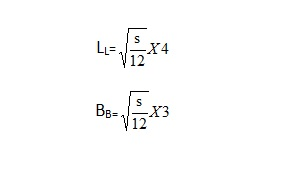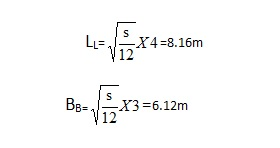LED显示解决方案一站式品牌供应商 国家高新技术企业
18269000899 13077789478

# LED显示屏安装，LED 显示屏箱体计算方式

LED显示屏经过多年的发展慢慢取代了投影产品成为企事业单位的形象推广，产品展示会议显示屏的必备品，由于LED全彩显示屏是由多张显示模组拼接而成的，而且室内LED全彩屏及室外LED大屏的显示模组规格很多，常常有客户咨询做一个LED全彩屏规格是怎么确定的？怎么计算的？我们总结以下方式方法。

1、室内LED显示屏模组箱体计算方式

(1)给出LED显示屏的具体数据(长、宽，面积)

MLBB= L1B1/L2B2

L1=5.8mx 1000÷320= 18.125≈18

B1= 2.6mx 1000÷160= 16.25≈16

LsBs=L2B2x LB

Ls=320x 18= 5760mm5.76m

Bs= 160x 16=2560mm2.56m

ε=k ×λ

g=(18x128)*(16x64)

(2) 只给出LED显示屏的面积，没有长宽。:LL=3.46mBL=2.60m

2室外LED显示屏的计算方式

(1)给出屏的具体数据(长、宽)

LXBX=L1B1/LDBD

LX= 10m x 1000÷768= 13.0208≈13

BX= 6m x 1000÷768=7.8125≈8

LSBS=LDBD×mn

LS= 768×13 =9984mm9.984m

BS=768 x8 =6144mm 6.144m

S=9.984x 6.144= 61.341696≈61.34㎡

ε=λXL X L1 XλXB X B1=128x 23 * 128x8

LED显示屏实际宽=16000/160=100=100×160=16m

LED显示屏实际高10000/160=62.5≈62×160 = 9.92m

LED显示屏面积为: 16x9.92= 158.72㎡

(2)只给出屏的面积，没有长宽。

实例2-3:制作P8的户外全彩LED显示屏的面积为: 50㎡ 若只给出了LED显示屏的面积，长宽需要计算。可以按长:宽比为4:316:9的比例计算，这样LED显示屏的画面显示效果好。以4:3为例计算LED显示屏的理论长和宽，即实例2-4:制作一块户外P8全彩LED显示屏，面积大概100。需要确定LED显示屏的实际宽和高，求出LED显示屏实际面积。但在计算前应确定是制作16:9 还是4:3LED显示屏。若选择制作16:9LED显示屏，则设显示屏宽为x，则显示屏高为9/16X

根据下式求出X

9/16X2= 100X=133334

LED显示屏宽13.333 4m，高为7.5m

LED显示屏宽13 333.4/256 = 52.08352

LED显示屏高7500/128 = 58.5959

LED显示屏实际高为59x 128= 7.552m

LED显示屏实际面积13.312x 7.552 = 100.53m2

3/4X2= 100X=11.546 8

LED显示屏宽为11.546 8m,高为8.660m

LED显示屏宽11 546.8/256 =45.145

LED显示屏实际宽为45x 256- 11.520m

LED显示屏高8660/128 = 67.65668

LED显示屏实际高为68x 128 8.704m

LED显示屏面积11.520 x 8.704- 100.27㎡。

【相关推荐】

18269000899
• 电话

18269000899